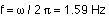## Resonance

11-22-99

Sections 10.5 - 10.6

### An example, using a mass on a spring

Here's a good problem that brings in everything we know about springs, plus all the new things we're doing on SHM. You have a block with a mass of 0.2 kg. You also have a spring, but you don't know the spring constant. When you hang the spring vertically and attach the block, the spring stretches by 9.8 cm (at equilibrium) . You then take the spring and mount it horizontally with the mass at one end, with the mass resting on a frictionless surface. You displace the mass by 5 cm from the equilibrium position and let it go from rest, causing the mass to oscillate back and forth in simple harmonic motion.

Some good questions to ask are:

(a) What's the frequency of the motion when the mass is oscillating?

(b) What's the maximum speed of the mass, and where does it reach this maximum speed?

(c) If the equilibrium position is x = 0, and you let it go from x = +5 cm, what is the position of the mass exactly two seconds after you let it go?

To find the frequency for a mass on a spring, we need to know the spring constant and the mass. The mass is given, and the spring constant can be found from the information obtained when you hung the spring and mass vertically. At equilibrium, the upward spring force, kx, must balance the weight, mg. This gives:

k = mg/x = 0.2(9.8) / 0.098 = 20 N/m

The angular frequency is the square root of k/m, so that's:The frequency is then:This corresponds to a period of 0.628 seconds.

When the mass is oscillating horizontally, the maximum speed can be found in two different ways. One is to use the fact that the maximum speed is simply the amplitude multiplied by the angular frequency. This gives:

vmax= 0.05 (10) = 0.5 m/s

Another method is to apply conservation of energy. When the mass was initially let go from rest, all the energy was stored as potential energy in the spring. This initial energy is:

PE = 0.5 kx2= 0.5 (20) (0.05)2 = 0.025 J

When the spring passes through the equilibrium position, there is no potential energy. All the energy is kinetic, and we haven't lost any energy. This allows us to calculate the speed at which it passes through equilibrium:

at equilibrium : KE = 0.5 mv2 = 0.025 J

Solving for the speed gives v = 0.5 m/s.

So, the maximum speed is 0.5 m/s, and the mass reaches this maximum speed at the equilibrium position, because that's the only place where the energy is entirely kinetic energy.

Now, what about the position of the mass at t = 2 seconds? All we need to do is to plug that time into the equation:One thing to be very careful of here is that the angular frequency is in radians/second, so you have to put your calculator in radians mode to do the cosine (or convert the radians to degrees). The amplitude, A, is 0.05 m, the amount we displaced the mass in the first place. This gives :

x = 0.05 cos (20 radians) = 0.0204 m, or just over 2 cm. The fact that this answer is positive means it's on the same side of the equilibrium position as the starting point.

One way to check this is to figure out how many periods are in 2 seconds. Dividing the time by the period gives 2 / 0.628 = 3.18 periods. So, the mass has gone back and forth completely 3 times. The extra 0.18 is the important bit. One quarter (0.25) of a period represents the motion from the endpoint to the equilibrium position. 0.18 is a little less than that, so it must be getting close to the equilibrium position but not be quite there yet. That's certainly consistent with it being at +2 cm.

### Damped harmonic motion

In the absence of any resistance forces (like friction and air resistance), most simple harmonic motions would go on unchanged forever. In reality, this doesn't happen, because there are resistance forces.

Damped harmonic motion - harmonic motion in which energy is steadily removed from the system.

There are three kinds of damping:

1. Critically damped - the damping is the minimum necessary to return the system to equilibrium without over-shooting.
2. Underdamped - less than critical, the system oscillates with the amplitude steadily decreasing.
3. Overdamped - More than critical, the system returns slowly towards equilibrium.

### Driven harmonic motion

Also known as forced harmonic motion, this is harmonic motion in which the system is given a periodic push. A perfect example is a person on a swing.

How the system behaves depends on how the frequency of the driving force compares to the natural frequency of oscillation of the system.

The most efficient way to transfer energy from the driver to the system is to match the frequency of the driving force to the natural frequency of the system, such as you do when pushing someone on a swing. This is known as resonance. At resonance, relatively small driving forces can build up to large-amplitude oscillations, just because energy is continually being injected into the system at just the right frequency.